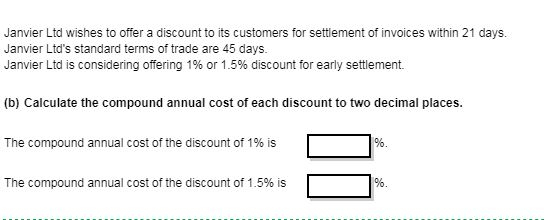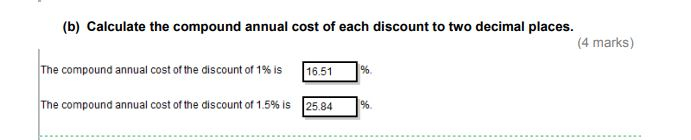# Compound annual cost

Hi,

Please can someone tell me how to work this out with a simple calculator or do i need a scientific calculator to work out the power of?

Thanks
Sophie

• Hi Sophie

Simple calculators won't let you do the power of directly. However you can do it by multiplying it by itself X times.

3^4 = 3*3*3*3

Hope this helps (is easier with a scientific calculator for sure)
AAT Level 4, MAAT
ACCA in progress
F4- Passed Aug 2020
F5- Passed Dec 2020
F6- Passed Sep 2020
F7- Passed June 2021
F8 - Passed Sep 2021
F9 - Passed June 2021

SBL -
SBR - Passed Mar 22
ATX - Passed Dec 21
APM - Passed June 22
• Pian32 said:

Hi Sophie

Simple calculators won't let you do the power of directly. However you can do it by multiplying it by itself X times.

3^4 = 3*3*3*3

Hope this helps (is easier with a scientific calculator for sure)

thank you so much for replying I've attached the question i was trying to work out, would it be possible to do this or no i'd need the correct calculator?
• Pian32 said:

Hi Sophie

Simple calculators won't let you do the power of directly. However you can do it by multiplying it by itself X times.

3^4 = 3*3*3*3

Hope this helps (is easier with a scientific calculator for sure)

thank you so much for replying I've attached the question i was trying to work out, would it be possible to do this or no i'd need the correct calculator?• I don't think this is in a module I completed. However looking at the formula for these types of questions and how it's calculated I don't think you can use a simple calculator. (As you'll have fractional powers)
AAT Level 4, MAAT
ACCA in progress
F4- Passed Aug 2020
F5- Passed Dec 2020
F6- Passed Sep 2020
F7- Passed June 2021
F8 - Passed Sep 2021
F9 - Passed June 2021

SBL -
SBR - Passed Mar 22
ATX - Passed Dec 21
APM - Passed June 22
• Pian32 said:

I don't think this is in a module I completed. However looking at the formula for these types of questions and how it's calculated I don't think you can use a simple calculator. (As you'll have fractional powers)

thank you so much for your help
• @Pian32 i now have the correct calculator but i still cannot get the answer ive been doing for eg 1/99 ^ 365/24 and i tried 1/99+1 ^ 365/24-1*100% but i still cannot get the same answer as above
• So the equation I think you want to use is

((1+ r/(100-r))^(365/days)) - 1 = compound cost

r = rate of discount
days = Normal day - discount day

Hope this helps
AAT Level 4, MAAT
ACCA in progress
F4- Passed Aug 2020
F5- Passed Dec 2020
F6- Passed Sep 2020
F7- Passed June 2021
F8 - Passed Sep 2021
F9 - Passed June 2021

SBL -
SBR - Passed Mar 22
ATX - Passed Dec 21
APM - Passed June 22
• Pian32 said:

So the equation I think you want to use is

((1+ r/(100-r))^(365/days)) - 1 = compound cost

r = rate of discount
days = Normal day - discount day

Hope this helps

thanks @Pian32 i think i'm just i'm just not getting i cannot get this answer thank you for explaining
• I noticed it simplifies slightly:

[(100/(100-r)) ^ (365/days)] - 1 = Compound cost

If we use the figures from the question.

a)
[ (100/99) ^ (365/24) ] -1 =Compound cost

[1.01010^15.208333] - 1 =Compound cost

b)
[1.0152284^15.208333]- 1

I think these get the answers you need. (I'd put the fractions in on the calculator as it's more accurate) I think this also gets the decimal not the %age so * 100 to convert to a percentage at the end.

If you're still not getting the answer what are you getting? Might be able to work backwards to find what's going wrong.
AAT Level 4, MAAT
ACCA in progress
F4- Passed Aug 2020
F5- Passed Dec 2020
F6- Passed Sep 2020
F7- Passed June 2021
F8 - Passed Sep 2021
F9 - Passed June 2021

SBL -
SBR - Passed Mar 22
ATX - Passed Dec 21
APM - Passed June 22
• Pian32 said:

I noticed it simplifies slightly:

[(100/(100-r)) ^ (365/days)] - 1 = Compound cost

If we use the figures from the question.

a)
[ (100/99) ^ (365/24) ] -1 =Compound cost

[1.01010^15.208333] - 1 =Compound cost

b)
[1.0152284^15.208333]- 1

I think these get the answers you need. (I'd put the fractions in on the calculator as it's more accurate) I think this also gets the decimal not the %age so * 100 to convert to a percentage at the end.

If you're still not getting the answer what are you getting? Might be able to work backwards to find what's going wrong.

thank you so much @Pian32 ive not manged to get the correct answers